## Articulo

•Citado por SciELO
•Accesos

•Similares en SciELO

## versión impresa ISSN 0186-1042

Ranking and optimal selection of investments with internal rate of return and benefit–cost ratio: a revision

Ordenamiento y selección óptima de inversiones con la tasa interna de retorno y la relación beneficio–costo: una revisión

Ignacio Vélez–Pareja

Universidad Tecnológica de Bolívar, Cartagena, Colombia. ivelez@unitecnologica.edu.co

Fecha de recepción: 25.01.2011.
Fecha de aceptación: 21.02.2011.

Abstract

The purpose of this paper is to present a procedure to include the implicit assumptions of Net Present Value (NPV) in the Internal Rate of Return (IRR), and the profitability index (benefit–cost ratio B/CR). The resulting indicators are the weighted IRR (WIRR) and the expanded B/CR (EB/CR). These two desirability measures are designed for variable and constant discount rates. They have the property to coincide with the NPV ranking for investment analysis and hence, will maximize value. Examples are presented.

Keywords: Net present value, NPV, internal rate of return, IRR, benefit–cost ratio, B/CR, profitability index, NPV assumptions.

JEL Classification: D92, E22, E31, G31

Resumen

El propósito de este trabajo es presentar un procedimiento para incluir los supuestos implícitos del valor presente neto (VPN) en la tasa interna de retorno (TIR) y el índice de rentabilidad (relación beneficio–costo RB/C). Los indicadores resultantes son la TIR ponderada (TIRpond) y la relación beneficio costo ampliada (RB/CAmp). Estas dos medidas de rentabilidad o deseabilidad de las inversiones están diseñadas para tasas de descuento variables y constantes; tienen la propiedad que al ordenar las alternativas de inversión con ellas ese ordenamiento coincide con el del VPN y, por tanto, maximizan el valor; se presentan algunos ejemplos.

Palabras clave: valor presente neto (VPN), valor actual neto (VAN), tasa interna de retorno (TIR), relación beneficio–costo (RB/C), índice de rentabilidad, supuestos del VPN.

Clasificación JEL: D92, E22, E31, G31

Introduction

The contradictions between Net Present Value, NPV, and the revered Internal Rate of Return, IRR and Benefit Cost Ratio B/CR1 have been well discussed in the financial literature by Lorie and Savage (1954), Grant and Ireson (I960), Fleischer (1966), Bacon (1977), Oakford, Baimjee and Jucker (1977), Canada and White (1980) and Beidleman (1984), just to mention some classical few. Magni (2010 has a complete relation of contributions to study this problem. The traditional procedure to solve this conflict is to calculate the incremental IRR and the incremental B/CR as presented by Grant and Ireson (1960), Bernhard (1989), Brealey and Myers (2003) and Berk and Demarzo (2009). However, this procedure is not easier to understand than NPV and the intuitive appeal of IRR and B/CR is a strong incentive for practitioners to keep using them. Martin2 (1995) presents an apology of the IRR. Others authors such as Baldwin (1959), Lin (1976), Athanasopoulos (1978), Beaves (1988) and Bernhard (1989) have proposed modified IRR's taking into account the reinvestment of intermediate cash flows. Velez–Pareja (1979a, 1979b, 1979c and 1994) presented an approach that modifies the IRR and the B/ CR, and takes into account the implicit and explicit assumptions in NPV, but In some isolated cases the method fails. Shull (1992) starts from the modified IRR proposed by Beaves (1988), Bernhard, (1989) and Lin (1976) to construct the adjusted overall rate of return. Biondi (2006) traces back the idea of reinvestment of intermediate cash flows to around 18th century with the work of Duvillard (1787). Magni, (2010) proposes the Average Internal Rate of Return, which has the virtue that complex–valued numbers and all the usual problems of IRR disappear. Walker, Check, and Randall, (2010) discuss on the inconsistency in teaching these decision tools.

This paper criticizes a wrong assumption regarding cash flows: many consider they are funds available for the firm3 and that remain within the firm. It also studies, criticizes and modifies the IRR method proposed by Shull and suggests a method to adjust the B/CR in order to make them coincide with the NPV ranking. A formal proof is presented for both of them. It is necessary to make NPV, IRR and B/CR compatible by the explicit inclusion of the implicit assumptions of NPV in the IRR and B/CR. The expanded benefit–cost ratio is presented and both methodologies are illustrated with examples to show how consistent they are with shareholder wealth maximization.

The paper is organized as follows: Initially the paper discusses the implicit assumptions of decision methods and what is called the overall rates of returns. Next, it discusses the proposed procedures which are illustrated with numerical examples and discusses the importance and drawbacks of using relative measures. Finally, it concludes.

Implicit assumptions in investment decision methods

The contradictions between NPV, IRR and B/CR rankings have their origin in the different assumptions of these three methods. These assumptions are: the reinvestment of intermediate cash flows, and the amount to be invested in each alternative NPV assumes implicitly reinvestment of intermediate cash flows and investment of the extra investment amount at the discount rate used to calculate NPV; IRR assumes implicitly reinvestment at the same IRR and is silent regarding the size of investment; B/CR assumes implicitly reinvestment as in the case of NPV calculation and also is silent regarding the size of investment.

The idea in this paper is to include explicitly both assumptions in the IRR and B/CR calculations.

The Reinvestment of Intermediate Cash Flows

Textbooks include net cash flows or free cash flows when calculating the present value in a firm and it might be understood as if flows were the net result of adding revenues and subtracting expenses and this might give the wrong idea that cash flows are funds available to the firm. For example, when shown how to calculate the NPV of a firm they usually say, it is the present value of positive net cash flows minus the present value of negative net cash flows

Funds contributed to the firm come from shareholders and debt owners. The free cash flow belongs to them. Therefore, the reinvestment assumption is related to what the owners of cash flows do with those cash flows and not related to what the firm does with them. Hence, this implicit assumption says that shareholders and debt owners reinvest what they receive from the firm at their own opportunity cost or discount rate. For example, assume that debt is provided by a bank. When the bank receives back the money it has lent to a firm it lends again that money to its customers and, if economic conditions have not changed, at the same rate at which it lent to the firm. Shareholders do something similar. Its expected rate of return, the cost of equity would not change if economic conditions remain the same, and it is reasonable to expect the shareholder will invest her money at least at her opportunity cost. The well–known weighted average cost of capital, WACC is the result of weighting these two opportunity costs.

In the case of a project within a firm it is the same. The firm receives initially all the cash flows and finance the project with a mixture of cost of debt and cost of equity and uses the WACC as its hurdle rate. The firm then invest those cash flows in other projects where at least the WACC is the hurdle rate. In this case, the firm acts as the sole shareholder in the project. Finally, benefits from the project will be received as cash flows to the firm's shareholders.

Hence, it is clear that free cash flow of a firm is NOT what is left in the firm. Free cash flows are the funds available and paid to share and debt holders. It might sound paradoxical, but the greater the cash flows paid to debt and shareholders, the greater the firm value and its NPV. In summary: the reinvestment of cash flows assumed in the calculation of the NPV and B/CR is not done by the firm, but by the owners of equity and debt. Finally, the free cash flow that is drawn in the cash flow diagram is not the result of subtracting revenues less expenses, but what is paid to shareholders and owners of debt.

On the other hand, the IRR assumes implicitly that the reinvestment of intermediate cash flows is made at a rate equal to the same IRR. This is, once debt and shareholders receive the cash flow, both of them reinvest it in another and identical firm with the same IRR. This only might happen with the bank when providing loans to a firm. A counter argument might be that when the firm does not distribute (and this implies not to pay to debt holders would mean bankruptcy) it is equivalent to reinvest at IRR. In the best case, nothing grants that those funds are invested at the same IRR. In general, excess cash is kept as cash or invested in market securities and this originates the well–known agency problem of the cost of free cash flow noted by Jensen (1986).

A word has to be said on why to include the assumptions of the NPV in the IRR and the B/CR and not the contrary. First, NPV measures the value created by an alternative for the firm as Tham and Velez–Pareja, (2004) and Velez–Pareja, (2000) suggest and NPV is what is left after the cash flow has turned back the investment and the cost of capital. IRR is a relative measure of the amount of resources generated by the amount invested (this is not a measure of the value generated by the alternative) and the B/CR measures how many times the alternative generated the amount invested (it is not either a measure of generated value). Second, the assumption to reinvest the cash flows at the rate of discount is more plausible than to reinvest the cash flows at the IRR. The discount rate is defined from actual interest rates available in the market (the cost of debt and the cost of equity as proposed by, Tham and Velez–Pareja, (2004). The IRR is inherent to a given cash flow profile and not necessarily is available for the investor during the planning horizon.

The amount to be invested in each alternative

When analyzing mutually exclusive alternatives it is assumed that there exist funds to invest in any of the alternatives considered as noted by Athanasopoulos (1978).

This means that the firm has available the funds needed even for the "largest" alternative. Then, there is a hidden assumption regarding the investment of the difference between the present value of the investment in the actual alternative and the alternative with the largest present value of the amount invested. This implicit assumption usually is not mentioned in the literature. The usual assumption mentioned in most finance textbooks is the reinvestment of intermediate cash flows at the discount rate, when NPV calculations are made. In other words, every time a "smaller" alternative is analyzed, there will be some funds in excess. This means that each alternative could be seen as composed of two cash flows: one the cash flow associated to the alternative itself and a second one, the cash flow of the difference in the amount invested. This later cash flow is composed of two flows of funds: one outflow at the beginning (the difference in amount invested, K–I, where K is the total funds available in present value and I is the present value of the amount to be invested in the current alternative) and an inflow at period n equal to (K–I)(1+i)n. The NPV considers implicitly that those funds are invested at the rate of discount and its NPV is equal to 0.

On the other hand, as said, the IRR and the B/CR are silent about this difference. In fact, as relative measures, IRR and B/CR are blind to the amount invested. (Simply recall that you can get an IRR of 500% either if you invest \$1 today and receive \$6 in a year or if you invest \$1 million and receive \$6 millions in a year).

The NPV is an absolute, not a relative measure of the value added to the firm. This means that it takes into account the amount invested when calculating added value. In fact, the NPV subtract the amount invested from the present value of cash flows discounted at time 0. On the other hand, IRR tells if there is some value added, but it never says how much value has been created.

The overall rates of return

The assumptions included in the NPV are reasonable as discussed above. Hence, in order to construct an index that is consistent with NPV, the implicit assumptions of NPV has to be included explicitly in the procedure to be used. These two procedures are the Internal Rate of Return (IRR) and Benefit Cost Ratio (B/CR).

Athanasopoulos (1978), does not distinguish between negative or positive cash flows and assume that all of them are reinvested at the same discount rate used to calculate NPV.

On the other hand, Shull (1992) refers to the overall rates of return as the rate of return of a terminal value resulting from the intermediate cash flows compounded at the rate of discount and an investment base defined as the present value of the amounts invested through the life of the firm. He distinguishes two overall internal rates of returns: the first one, the Modified Internal Rate of Return (what he calls the MIRR method4) that implies that any positive cash flow followed by a negative cash flow, will be used to fund the negative cash flow. In this case, the terminal value is the future value of all positive net cash flows after funding for any negative cash flow that occurs in the future and the firm investment base is the present value of any negative net cash flow, after being funded by the positive cash flows. Shull (1992) assumes that positive cash flows could finance negative cash flows. This is not what happens in reality. As said above, free cash flows are paid out to share and debt holders. These funds are out of the firm and if in the next period appear that there is a deficit (a new investment with a negative cash flow) that deficit has to be financed that period and certainly not with previous cash flows that now are in the hands of equity and debt owners. The argument posited above on the destiny of free cash flows in a firm clearly says that assuming that a positive FCF will fund a negative cash flow in the following period is counter evident: positive cash flows are received by owners of capital; hence those flows are no longer available by the firm and cannot be assumed to fund any future outflow.

As said above, the cash flows (free cash flows) generated by an investment are outflows from the firm toward the owners of debt and equity. Hence, it makes no sense to assume that positive cash flows fund the negative cash flows (investment). It is ontologically impossible because those cash flows do not remain in the firm.

The terminal value at period n is, in generalWhen discount rate is constant, then we have a very special case as follows:The second overall rate of return (what Shull calls the IRR* method) considers as terminal value the future value of the net positive cash flows (without funding the intermediate negative cash flows) compounded at the discount rate and the investment firm base is the present value of all negative net cash flow (without any funding from the positive net cash flows). In the first case the interpretation of the MIRR is the yield produced by the net investment of external funds. In the second case the interpretation of the IRR* is the yield produced by the total firm investment. Shull advocates for the MIRR as the correct one.

For consistency with the idea of cash flows paid to owners of debt and equity and value generated by the firm as Tham and Velez–Pareja, (2004) mention, in this paper the IRR* is considered the correct procedure and not the MIRR as defined and proposed by Shull (1992).

In general, an overall rate of return, ORR, is defined byWhere ORR is the overall rate of return, TV is the terminal value and is the amount compounded of the net positive cash flows at the discount rate, IB is the investment base and is the present value of all negative net cash flow at the same discount rate and n is the horizon period for the firm.

In general, TV as defined for (2) is equation (1a) when F is positive. IB will be defined as followsWhen there are variable discount rates any IRR approach has a serious drawback: there is no unique discount rate to compare IRR with. The same happens with any modified IRR as we deal with in this work. One possible solution is to consider the cumulated IRR and compare with the cumulated discount rate factor. In other words, instead of calculating an IRR per period we would calculate the IRR for the whole planning horizon, and compare that with the cumulated discount rate for the planning horizon. For instance,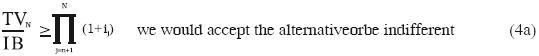Proposed procedures

In order to construct consistent procedures for investment decision making it is necessary to include the assumptions discussed in the previous section in the two popular methods: the IRR and the B/CR.

The weighted internal rate of return (WIRR)

In order to include the first assumption of NPV in IRR the reinvestment assumption has to be made explicit in the cash flow and recalculate the IRR as indicated by the ORR, above. This is, all the inflows will be compounded at the rate of discount up to period n and all the outflows will be discounted at the discount rate to instant 0. This will convert the original cash flow of the firm into a cash flow with an investment outlay at instant 0 and an inflow at period n. This cash flow defines a modified rate of return (IRR* defined above or the internal rate of return with reinvestment).

Including the second assumption means to find the difference between the present value of all the outflows as calculated in the previous paragraph and the maximum outflow in the collection of alternatives. This amount will be compounded up to period n at the discount rate. This cash flow (an outflow at instant 0 equal to the difference in initial investment and an inflow of that difference compounded at the discount rate at period n) defines an IRR equal to the discount rate. This means that the NPV of this extra investment at the discount rate is zero.

The sum of the two cash flows will define an overall rate of return. This overall rate of return is the weighted internal rate of return (WIRR).

Then, the new rate of return is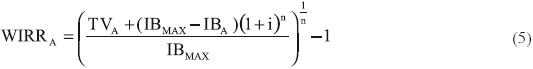Where WIRRA is the weighted internal rate of return, TVA is the compounded value at the discount rate of inflows, IBMAX is the maximum present value of the amount invested in the group of alternatives and IBA is the amount invested in the alternative under study, n is the number of periods and i is the discount rate.

A simple proof that WIRR is consistent with accept/reject decisions with NPV and its ranking is provided.

Let firm A has a larger investment amount than firm B, this is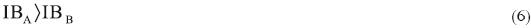Then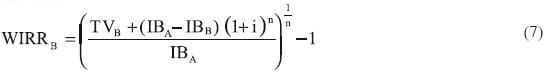and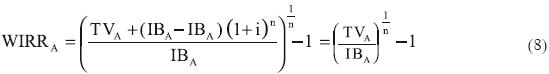If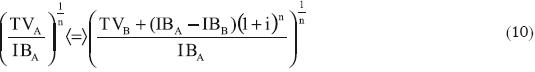andandQED.

This proof is also valid for the case when the investment in B is larger than the investment in A. From this proof the transitivity of WIRR can be deduced.

In the same vein, when we only consider the Modified Internal Rate of Return (à la Excel) MIRR or IRR* using Shull notation, it can be shown that in general, it does not produce the same ranking as the NPV does and might not select the optimal alternative, as follows:

Let firm A has a larger investment amount than firm B, this isThen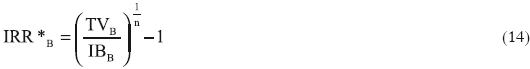and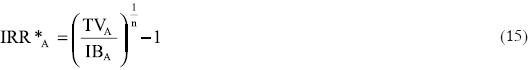If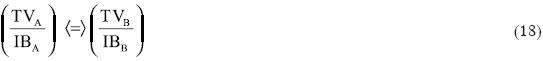If NPVA = NPVB the ranking would favor alternative B (IBA>IBB). Hence, the ranking using IRR* is not, in general, the same as the ranking by NPV. QED.

Example 1

Assume the general case for variable discount rates. Now we show an example where the method is used to solve the problem of contradictions between NPV and IRR with variable discount rates.5

Observe that IRR* (or correct MIRR) does not solve the contradiction. This is understandable because IRR* does not include the second implicit assumption of NPV: this is the investment of the excess amount at the discount rate.

As can be seen from tables 1 and 2 TV/IB > cumulated discount factor. Hence, both projects are desirable.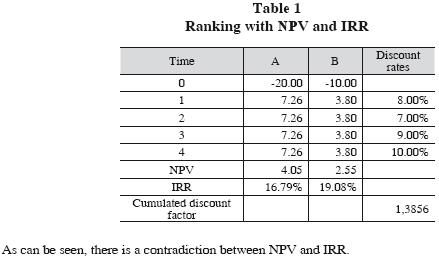Example 2

Consider four mutually exclusive alternatives and a discount rate of 6% per annum. (This is the same example presented by Shull (1992)).In this case a contradiction in the optimal selection of alternatives is found between NPV and IRR. Alternative C has the highest NPV, but alternative B has the highest IRR. The NPV ranking is C>B>D>A. The IRR ranking is B>C>A>D.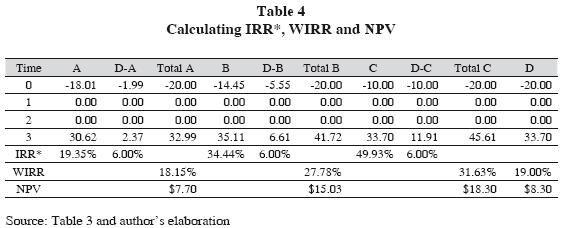Observe that the NPV for the four alternatives is the same as the calculated with the original cash flows in table 3. The ranking with NPV and WIRR is the same: C>B>D>A.Observe that the IRR* does not give the same ranking as the NPV. The rankings with NPV and WIRR are the same: C>B>D>A.

As a byproduct, this method eliminates the problem of multiple rates of returns. Teichroew, Robichek and Montalbano (1965a, 1965b) and more recently Hazen (2003) and Magni (2010) studied the problem of multiple IRR using relatively more complex algorithms.

The expanded benefit cost ratio (EB/CR)

When analyzing mutually exclusive alternatives it is common use to calculate the benefit cost ratio or profitability index. As mentioned above, this indicator is a relative one and does not take into account the size of the investment. The profitability index might generate inconsistencies in the rankings as compared with NPV rankings, as well. As was done with the IRR, the implicit assumptions found in the NPV model have to be included in B/CR calculations.

By definition, B/CR considers the present value of inflows divided by the present value of outflows when discounting the cash flow at the discount rate, the reinvestment at that rate is implicitly assumed in the calculation. The only adjustment to do is to include the present value of the inflow and the outflow of the additional cash flow. This is, the cash flow composed by the difference of the present values of the amounts invested (IBA–IBB, in the case of investments A and B and A>B) and present value of the compounded value of this difference. The present value of the inflow and the outflow is the difference (IBA–IBB) calculated for instant 0. Given this definition, the EB/CR is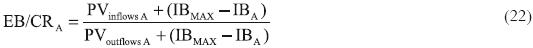where EB/CRA is the expanded benefit cost ratio, PV is the present value at the discount rate of the absolute value of inflows or outflows, IBMAX is the maximum present value of the amount invested in each alternative and IBA is the present value of the amount invested (outflows) in the alternative under study.

The proof that EB/CR is consistent with accept/reject decisions and with NPV ranking is simple.

Let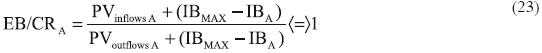then,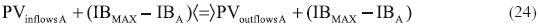andQED.

On the other hand, for proofing the consistency with NPV rankings, if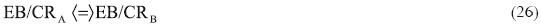but,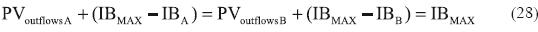then,and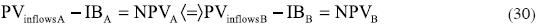QED.

In the same fashion it can be shown that B/CR does not always select the optimal investment decision under mutually exclusive alternatives.

Example 2

Working the same example 2,In this case there is not a contradiction between NPV and B/CR when selecting the optimal alternative. However, the NPV ranking is C>B>D>A, while the B/CR ranking is C>B>A>D.The calculations are, for alternative A: Present value of inflows forPresent value of extra amount invested IBa= PVoutflows A = 10 + 9/(1+6%)2 = 18.01 Maximum amount invested IBMAX = 20

IBMAX – IBA = 1.99The ranking by EB/CR is C>B>D>A and coincides with the NPV ranking.

A summary for example 2 and according to the summary presented for example 1, follows:As can be seen, the EB/CR and the B/CR with the cash flows from example 1, maintain the same rankings as NPV and IRR* respectively as in table 5. In particular, the EB/CR is consistent with the NPV ranking.

Ochoa (1987) and Echeverri (1987) conducted a simulation to test this indicator and there was 100% coincidence with NPV rankings.

The importance of relative measures

Someone might ask with reason, if academics know that NPV is superior to IRR and B/CR, why expending so much effort and time working out solutions like those presented above? The reason is simple: relative measures such as IRR and B/CR are widely used and they have an intuitive appeal that apparently makes them appear as more ease to understand. Teachers should teach all those methods, of course. However, they should warn students about the potential flaws and features of each of them. However, some teachers and textbooks on project appraisal suggest strongly the calculation of the NPV and the IRR and the B/CR. This is, they suggest that the analyst presents at the same time the collection of the three measures: NPV, IRR and B/CR. When those teachers are asked why to teach the student to work professionally with the three methods at the same time, the answer is "just to be sure that the decision is the correct one" (!). Imagine what could happen when an analyst presents a set of mutually exclusive alternatives with the NPV and the IRR and the B/CR and the recommendation is to select the firm with the highest NPV, but with a non–optimal IRR or B/CR. Probably more than one of the directors will ask why to choose an alternative that does not yield the highest return. Probably the analyst will be fired! The WIRR and the EB/CR could be a good option to avoid embarrassing situations like this one.

Concluding remarks

This paper presents two alternative methods for relative indicators (IRR and B/CR) that present inconsistencies with the NPV ranking of alternatives when mutually exclusive firms are studied. The first is the weighted internal rate of return, WIRR. The second one is the expanded benefit cost ratio, EB/CR or profitability index. Both methods, WIRR and EB/CR are consistent with NPV accept/reject criteria and NPV ranking. with WIRR the problem of multiple IRR's is overcome.

In addition, we present the two methods for constant and for variable discount and reinvestment rates. In the case of the WIRR, we find the same difficulties as with the simple IRR when having variable discount rates. when it is the case, the IRR and WIRR might present the same drawback: this is, nobody could guarantee that the IRR or WIRR will be higher than (or lower than) all the variable discount rates when using the WIRR or IRR for accept/reject decisions. In reality what would happen is that for some periods IRR and WIRR will be higher than some discount rates and for the rest will be lower. We have to keep in mind that IRR in any of its forms is just an average. we propose an approach to accept/reject decisions with the WIRR when there are variables discount rates.

However, the WIRR with variable discount rates will work for selection of mutually exclusive alternatives in the same fashion as it does for the special case of constant discount rate.

Methods like these are alternatives for those that prefer the firm analysis and appraisal based on IRR or B/CR.

References

Athanasopoulos, P.J. (1978). A Note on the Modified Internal Rate of Return and Investment Criterion. The Engineering Economist 23 (2): 131–133.         [ Links ]

Bacon, P.W. (1977). The Evaluation of Mutually Exclusive Investments. Financial Management, Summer (6): 55–58.         [ Links ]

Baldwin, H.R. (1959). How to assess Investment Proposals. Harvard Business Review 37 (May–June): 98–104.         [ Links ]

Beaves, R. G. (1988). Net Present Value and Rate of Return: Implicit and Explicit Reinvestment assumptions. The Engineering Economist, Summer (33): 275302.         [ Links ]

Beidleman, C.R. (1984). Discounted Cash Flow Reinvestment Rate Assumptions. The Engineering Economis 29 (2): 127–137.         [ Links ]

Bernhard, R. H. (1989). Base Selection for Modified Rates of Return and its Irrelevance for Optimal Firm Choice. The Engineering Economist (35): 55–65.         [ Links ]

Berk, J. and P. De Marzo (2009). Corporate Finance. The Core. Boston: Pearson.         [ Links ]

Biondi, Y. (2006). The double emergence of the modified internal rate of return: The neglected financial work of Duvillard (1755–1832) in a comparative Perspective. The European Journal of the History of Economic Thought 13 (3): 311–335.         [ Links ]

Brealey, R. A. and S. C. Myers (2003). Principles of Corporate Finance. 7th ed., Boston: McGraw–Hill–Irwin.         [ Links ]

Canada, J.R. and J.A. White, Jr. (1980). Capital Investment Decision Analysis for Management and Engineering. Englewood Cliffs, N.J.: Prentice Hall Inc.         [ Links ]

Duvillard de Durand, E.–E'. (1787). Recherches sur les rentes, les emprunts et les remboursemens [Researches on Rents, Loans and Repayments]. Paris et Genève: Franç. Dufart Imprimeur–Libraire. Cited by Biondi, (2006).         [ Links ]

Echeverri, E. (1987). Modelo aleatorio para ordenar alternativas de inversión. Monografía de investigación. Magister en Administración (MBA), (unpublished), Bogotá: Universidad de los Andes.         [ Links ]

Fleischer, G.A. (1966). Two Major Issues Associated with the Rate Return Method for Capital Allocation: The Ranking Error and Preliminary Selection. The Journal of Industrial Engineering 17 (4): 202–208.         [ Links ]

Grant, E.L. and W.G. Ireson (1960). Principles of Engineering Economy. 4th ed., New York: The Ronald Press Co.         [ Links ]

Hazen, G.B. (2003). A new perspective on multiple internal rates of return. The Engineering Economist 48 (1): 31–51.         [ Links ]

Jensen, M. C. (1986). Agency Cost of Free Cash Flow, Corporate Finance, and Takeovers. American Economic Review 76 (2): 323–329.         [ Links ]

Lin, S. A. (1976). The Modified Internal Rate of Return and Investment Criterion. The Engineering Economist 21 (Summer): 237– 247.         [ Links ]

Lorie, J.H. and L. J. Savage (1955), Three Problems in Rationing Capital. Journal of Business 28 (4): 229–239. Reproduced in Solomon, E. (Ed.), (1959). The Management of Corporate Capital. Glencoe, IL: The Free Press of Glencoe.         [ Links ]

Machain, L. (2005). Funciones financieras adicionales para Microsoft Excel®. Unpublished paper. Available at http://cashflow88.com/decisiones/funciones_financieras.pdf        [ Links ]

Magni, C. A. (2010). Average Internal Rate of Return and Investment Decisions: A New Perspective. The Engineering Economist 55 (2): 150–181.         [ Links ]

Martin, R. (1995). Internal Rate of Return Revisited, Social Science Research Network, Available at http://papers.ssrn.com/paper.taf?ABSTRACT_ID=39520.         [ Links ]

My Stock Market Power (2010). Available at http://www.mysmp.com/fundamental–analysis/benefit–cost–ratio.html.         [ Links ]

Oakford, R.V., S.A. Baimjee and J.V. Jucker (1977). The Internal Rate of Return the Pseudo–Internal Rate of Return and NPV and their Use in Financial Decision Making. The Engineering Economist 21 (3): 187–202.         [ Links ]

Ochoa, L. F. (1987). Dos casos sobre Evaluación de Proyectos, Monografía de Investigación. Magister en Administración (MBA), (unpublished). Bogotá: Universidad de los Andes.         [ Links ]

Shull, D. M. (1992). Efficient Capital Project Selection Through a Yield–Based Capital Budgeting Technique. The Engineering Economist 38 (1): 1–18.         [ Links ]

Teichroew, D., A.A. Robichek and M. Montalbano (1965a). Mathematical analysis of rates of return under certainty. Management Science 11 (3): 395–403.         [ Links ]

–––––––––– (1965b). An analysis of criteria for investment and financing decisions under certainty. Management Science 12 (3): 151–79.         [ Links ]

Tham, J. and I. Vélez–Pareja (2004). Principles of Cash Flow Valuation: An Integrated Market–Based Approach. Boston: Academic Press.         [ Links ]

Vélez–Pareja, I. (1979 a). Decisiones de inversión: textos complementarios, Industrial Engineering Department, Bogota: Universidad de los Andes (mimeo, 209 pp.         [ Links ]).

–––––––––– (1979b) Decisiones de inversión: textos preliminares, Industrial Engineering Department. Bogotá: Universidad de los Andes August (mimeo, 116 pp.         [ Links ]).

–––––––––– (1979c). Toma de decisiones, alternativas de decisión y algunos métodos para su ordenamiento y selección, Industrial Engineering Department, Bogotá: Universidad de los Andes (mimeo, 48 pp.         [ Links ]).

–––––––––– (1994). Evaluación financiera de proyectos de inversión. Bogotá: Superintendencia de Subsidio Familiar:        [ Links ]

–––––––––– (2000). Value Creation and its Measurement: A Critical Look at EVA. Cuadernos de Administración, June, (22): 7–31. Available at Social Science Research Network, http://papers.ssrn.com/paper.taf?ABSTRACT_ID=163466.         [ Links ]

–––––––––– (2006). Decisiones de inversión. Para la valoración financiera de proyectos y empresas, 5ª ed., Bogotá: Editorial Universidad Javeriana.         [ Links ]

Walker, J. S., H. F. Check, Jr., and K. L. Randall (2010). Does the Internal Rate of Return Calculation Require a Reinvestment Rate Assumption? There is Still No Consensus. ABE/FEA 11th Annual Conference, San Antonio. PROCEEDINGS 2010 Academy of Business Education. http://www.abe.sju.edu/check.pdf        [ Links ]

Notas

1 The benefit–cost ratio (also called profitability index) is defined as the ratio between the present value of net cash inflows and the present value of net cash outflows. The concept of benefit cost ratio was first introduced by Jules Dupuit, an engineer from France, in 1848. Later, a British economist, Alfred Marshall, further enhanced the formula that became the basis for benefit cost ratio. (http://www.mysmp.com/fundamental–analysis/benefit–cost–ratio.html, retrieved November 19, 2010)

2 Independent Contractor & Northwest Vista College, San Antonio.

3 From now and afterwards firm means either a company or a company with a new project.

4 It has to be said that this MIRR is different to the MIRR that is used in MS. Excel©. The later one is consistent with the IRR* proposed by Shull (1992).

5 Machain (2005) upon request from author and based upon Vélez–Pareja, (2006), developed some add–ins for Excel® and among them is included the WIRR with variable rates. They are available at http://cashflow88.com/decisiones/funciones_financieras.xla. See reference for user's instructions.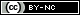Todo el contenido de esta revista, excepto dónde está identificado, está bajo una Licencia Creative Commons